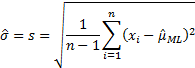/

### Maximum Likelihood Estimation of Normal Distribution

The probability density (likelihood) of a single variable drawn from a Normal distribution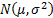is: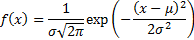Thus the likelihood ofindependent draws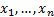from this distribution is: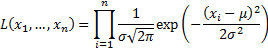To identify the values of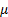and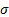that maximise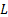it is easiest to identify the maximum of the log likelihood (as the two will be maximised for the same values ofandsince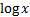is a monotonically increasing function of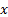). The log likelihood is: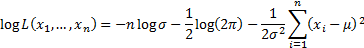This is maximised when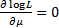and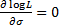, i.e. when: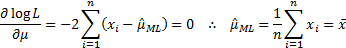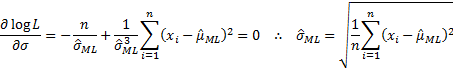The maximum likelihood estimator,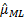, of the meanis thus the average of the observations,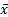. It is possible to show that this is also the minimum variance unbiased estimator of. The maximum likelihood estimator,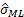, ofis the population standard deviation,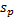of the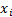which can be determined using the MnPopulationStdev web function. Please note that whilstis an unbiased estimator of,is a biased estimator of. The minimum variance unbiased estimator of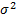is the sample variance (i.e. square of the sample standard deviation). The sample standard deviation is: